# lucidrains / vit-pytorch

Implementation of Vision Transformer, a simple way to achieve SOTA in vision classification with only a single transformer encoder, in Pytorch

Geek Repo

Github PK Tool## Vision Transformer - Pytorch

Implementation of Vision Transformer, a simple way to achieve SOTA in vision classification with only a single transformer encoder, in Pytorch. Significance is further explained in Yannic Kilcher's video. There's really not much to code here, but may as well lay it out for everyone so we expedite the attention revolution.

For a Pytorch implementation with pretrained models, please see Ross Wightman's repository here.

The official Jax repository is here.

A tensorflow2 translation also exists here, created by research scientist Junho Kim! 🙏

## Install

`\$ pip install vit-pytorch`

## Usage

```import torch
from vit_pytorch import ViT

v = ViT(
image_size = 256,
patch_size = 32,
num_classes = 1000,
dim = 1024,
depth = 6,
mlp_dim = 2048,
dropout = 0.1,
emb_dropout = 0.1
)

img = torch.randn(1, 3, 256, 256)

preds = v(img) # (1, 1000)```

## Parameters

• `image_size`: int.
Image size. If you have rectangular images, make sure your image size is the maximum of the width and height
• `patch_size`: int.
Number of patches. `image_size` must be divisible by `patch_size`.
The number of patches is: ` n = (image_size // patch_size) ** 2` and `n` must be greater than 16.
• `num_classes`: int.
Number of classes to classify.
• `dim`: int.
Last dimension of output tensor after linear transformation `nn.Linear(..., dim)`.
• `depth`: int.
Number of Transformer blocks.
• `heads`: int.
• `mlp_dim`: int.
Dimension of the MLP (FeedForward) layer.
• `channels`: int, default `3`.
Number of image's channels.
• `dropout`: float between `[0, 1]`, default `0.`.
Dropout rate.
• `emb_dropout`: float between `[0, 1]`, default `0`.
Embedding dropout rate.
• `pool`: string, either `cls` token pooling or `mean` pooling

## Simple ViT

An update from some of the same authors of the original paper proposes simplifications to `ViT` that allows it to train faster and better.

Among these simplifications include 2d sinusoidal positional embedding, global average pooling (no CLS token), no dropout, batch sizes of 1024 rather than 4096, and use of RandAugment and MixUp augmentations. They also show that a simple linear at the end is not significantly worse than the original MLP head

You can use it by importing the `SimpleViT` as shown below

```import torch
from vit_pytorch import SimpleViT

v = SimpleViT(
image_size = 256,
patch_size = 32,
num_classes = 1000,
dim = 1024,
depth = 6,
mlp_dim = 2048
)

img = torch.randn(1, 3, 256, 256)

preds = v(img) # (1, 1000)```

## Distillation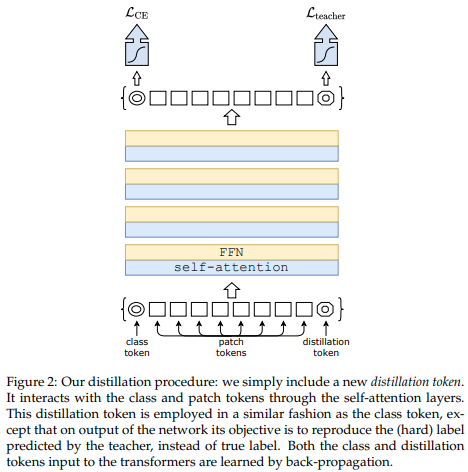A recent paper has shown that use of a distillation token for distilling knowledge from convolutional nets to vision transformer can yield small and efficient vision transformers. This repository offers the means to do distillation easily.

ex. distilling from Resnet50 (or any teacher) to a vision transformer

```import torch
from torchvision.models import resnet50

from vit_pytorch.distill import DistillableViT, DistillWrapper

teacher = resnet50(pretrained = True)

v = DistillableViT(
image_size = 256,
patch_size = 32,
num_classes = 1000,
dim = 1024,
depth = 6,
mlp_dim = 2048,
dropout = 0.1,
emb_dropout = 0.1
)

distiller = DistillWrapper(
student = v,
teacher = teacher,
temperature = 3,           # temperature of distillation
alpha = 0.5,               # trade between main loss and distillation loss
hard = False               # whether to use soft or hard distillation
)

img = torch.randn(2, 3, 256, 256)
labels = torch.randint(0, 1000, (2,))

loss = distiller(img, labels)
loss.backward()

# after lots of training above ...

pred = v(img) # (2, 1000)```

The `DistillableViT` class is identical to `ViT` except for how the forward pass is handled, so you should be able to load the parameters back to `ViT` after you have completed distillation training.

You can also use the handy `.to_vit` method on the `DistillableViT` instance to get back a `ViT` instance.

```v = v.to_vit()
type(v) # <class 'vit_pytorch.vit_pytorch.ViT'>```

## Deep ViT

This paper notes that ViT struggles to attend at greater depths (past 12 layers), and suggests mixing the attention of each head post-softmax as a solution, dubbed Re-attention. The results line up with the Talking Heads paper from NLP.

You can use it as follows

```import torch
from vit_pytorch.deepvit import DeepViT

v = DeepViT(
image_size = 256,
patch_size = 32,
num_classes = 1000,
dim = 1024,
depth = 6,
mlp_dim = 2048,
dropout = 0.1,
emb_dropout = 0.1
)

img = torch.randn(1, 3, 256, 256)

preds = v(img) # (1, 1000)```

## CaiT

This paper also notes difficulty in training vision transformers at greater depths and proposes two solutions. First it proposes to do per-channel multiplication of the output of the residual block. Second, it proposes to have the patches attend to one another, and only allow the CLS token to attend to the patches in the last few layers.

You can use this scheme as follows

```import torch
from vit_pytorch.cait import CaiT

v = CaiT(
image_size = 256,
patch_size = 32,
num_classes = 1000,
dim = 1024,
depth = 12,             # depth of transformer for patch to patch attention only
cls_depth = 2,          # depth of cross attention of CLS tokens to patch
mlp_dim = 2048,
dropout = 0.1,
emb_dropout = 0.1,
layer_dropout = 0.05    # randomly dropout 5% of the layers
)

img = torch.randn(1, 3, 256, 256)

preds = v(img) # (1, 1000)```

## Token-to-Token ViT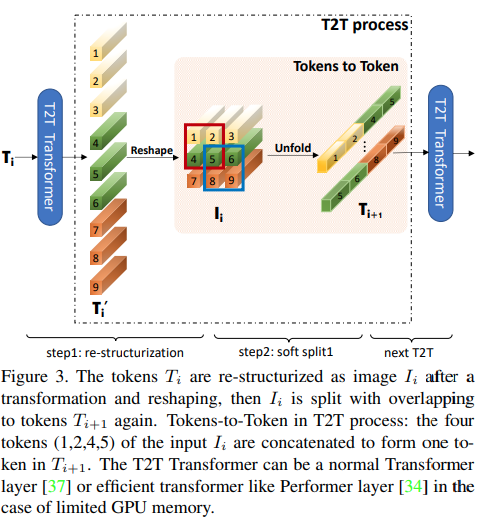This paper proposes that the first couple layers should downsample the image sequence by unfolding, leading to overlapping image data in each token as shown in the figure above. You can use this variant of the `ViT` as follows.

```import torch
from vit_pytorch.t2t import T2TViT

v = T2TViT(
dim = 512,
image_size = 224,
depth = 5,
mlp_dim = 512,
num_classes = 1000,
t2t_layers = ((7, 4), (3, 2), (3, 2)) # tuples of the kernel size and stride of each consecutive layers of the initial token to token module
)

img = torch.randn(1, 3, 224, 224)

preds = v(img) # (1, 1000)```

## CCT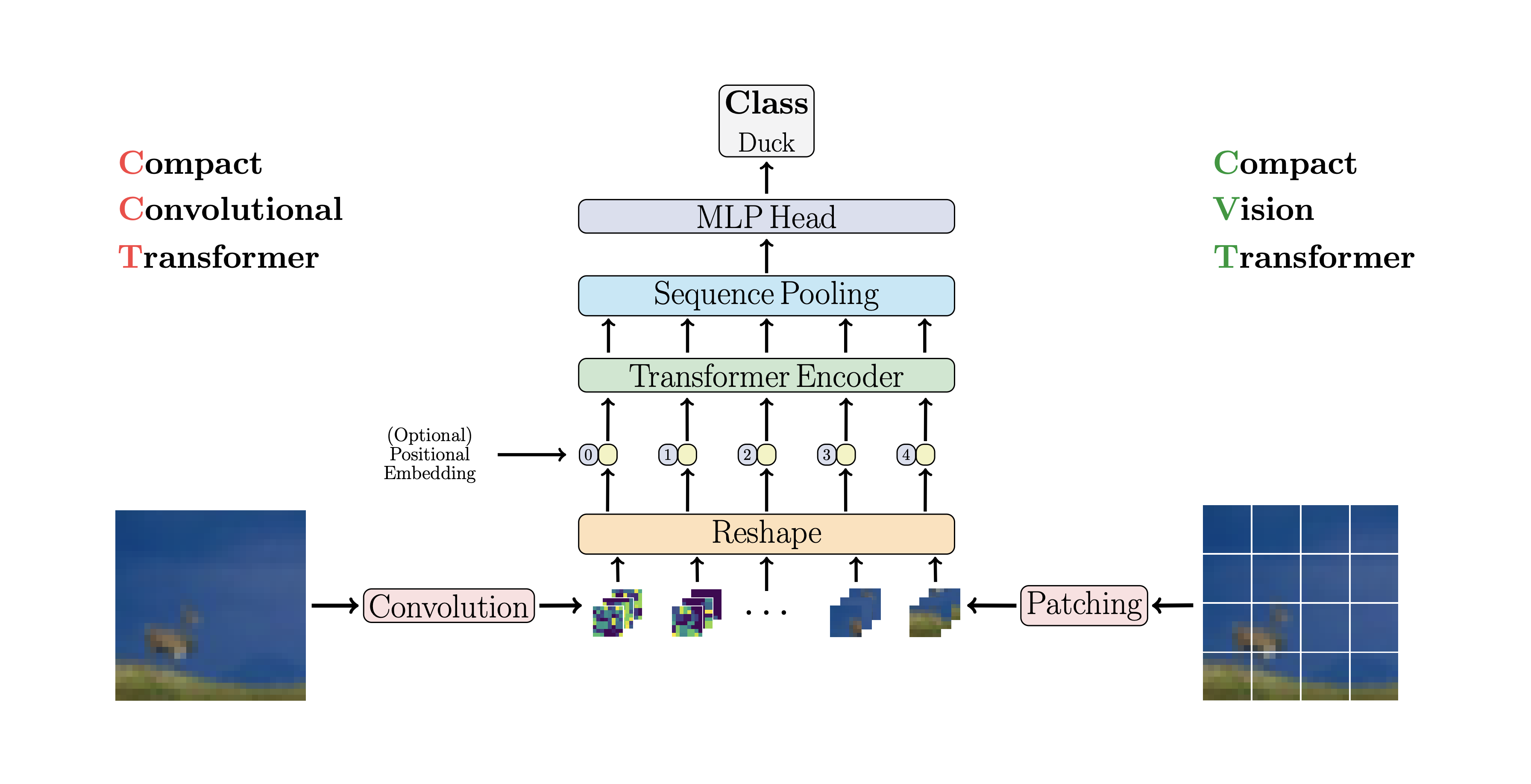CCT proposes compact transformers by using convolutions instead of patching and performing sequence pooling. This allows for CCT to have high accuracy and a low number of parameters.

You can use this with two methods

```import torch
from vit_pytorch.cct import CCT

cct = CCT(
img_size = (224, 448),
embedding_dim = 384,
n_conv_layers = 2,
kernel_size = 7,
stride = 2,
pooling_kernel_size = 3,
pooling_stride = 2,
num_layers = 14,
num_classes = 1000,
positional_embedding = 'learnable', # ['sine', 'learnable', 'none']
)

img = torch.randn(1, 3, 224, 448)
pred = cct(img) # (1, 1000)```

Alternatively you can use one of several pre-defined models `[2,4,6,7,8,14,16]` which pre-define the number of layers, number of attention heads, the mlp ratio, and the embedding dimension.

```import torch
from vit_pytorch.cct import cct_14

cct = cct_14(
img_size = 224,
n_conv_layers = 1,
kernel_size = 7,
stride = 2,
pooling_kernel_size = 3,
pooling_stride = 2,
num_classes = 1000,
positional_embedding = 'learnable', # ['sine', 'learnable', 'none']
)```

Official Repository includes links to pretrained model checkpoints.

## Cross ViT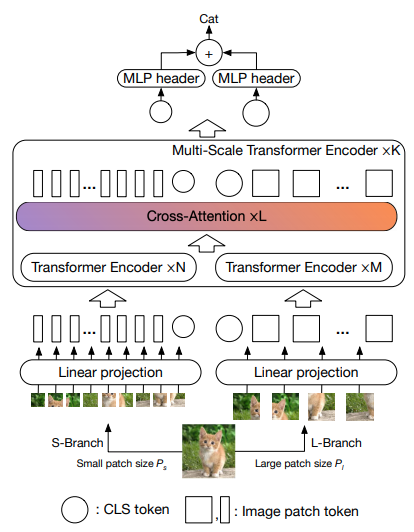This paper proposes to have two vision transformers processing the image at different scales, cross attending to one every so often. They show improvements on top of the base vision transformer.

```import torch
from vit_pytorch.cross_vit import CrossViT

v = CrossViT(
image_size = 256,
num_classes = 1000,
depth = 4,               # number of multi-scale encoding blocks
sm_dim = 192,            # high res dimension
sm_patch_size = 16,      # high res patch size (should be smaller than lg_patch_size)
sm_enc_depth = 2,        # high res depth
sm_enc_mlp_dim = 2048,   # high res feedforward dimension
lg_dim = 384,            # low res dimension
lg_patch_size = 64,      # low res patch size
lg_enc_depth = 3,        # low res depth
lg_enc_mlp_dim = 2048,   # low res feedforward dimensions
cross_attn_depth = 2,    # cross attention rounds
dropout = 0.1,
emb_dropout = 0.1
)

img = torch.randn(1, 3, 256, 256)

pred = v(img) # (1, 1000)```

## PiT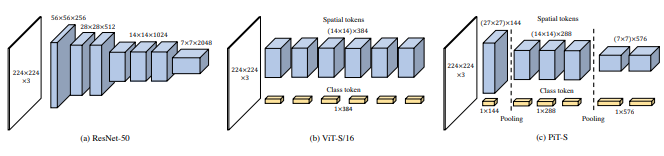This paper proposes to downsample the tokens through a pooling procedure using depth-wise convolutions.

```import torch
from vit_pytorch.pit import PiT

v = PiT(
image_size = 224,
patch_size = 14,
dim = 256,
num_classes = 1000,
depth = (3, 3, 3),     # list of depths, indicating the number of rounds of each stage before a downsample
mlp_dim = 2048,
dropout = 0.1,
emb_dropout = 0.1
)

# forward pass now returns predictions and the attention maps

img = torch.randn(1, 3, 224, 224)

preds = v(img) # (1, 1000)```

## LeViT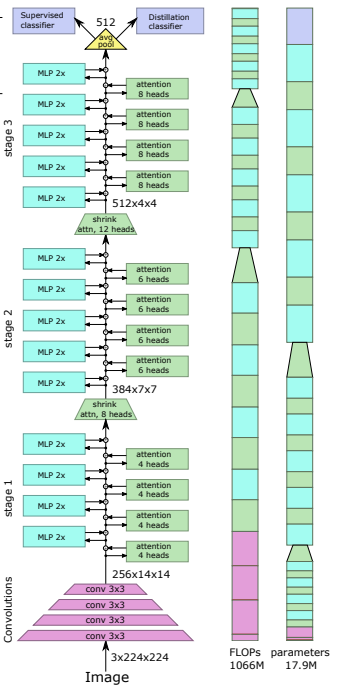This paper proposes a number of changes, including (1) convolutional embedding instead of patch-wise projection (2) downsampling in stages (3) extra non-linearity in attention (4) 2d relative positional biases instead of initial absolute positional bias (5) batchnorm in place of layernorm.

Official repository

```import torch
from vit_pytorch.levit import LeViT

levit = LeViT(
image_size = 224,
num_classes = 1000,
stages = 3,             # number of stages
dim = (256, 384, 512),  # dimensions at each stage
depth = 4,              # transformer of depth 4 at each stage
mlp_mult = 2,
dropout = 0.1
)

img = torch.randn(1, 3, 224, 224)

levit(img) # (1, 1000)```

## CvT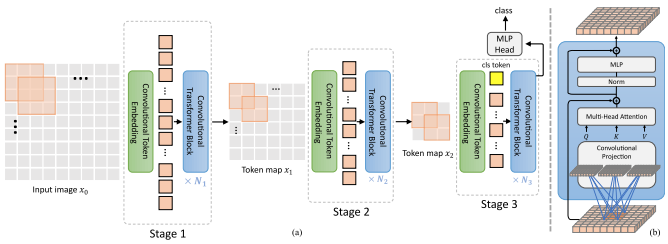This paper proposes mixing convolutions and attention. Specifically, convolutions are used to embed and downsample the image / feature map in three stages. Depthwise-convoltion is also used to project the queries, keys, and values for attention.

```import torch
from vit_pytorch.cvt import CvT

v = CvT(
num_classes = 1000,
s1_emb_dim = 64,        # stage 1 - dimension
s1_emb_kernel = 7,      # stage 1 - conv kernel
s1_emb_stride = 4,      # stage 1 - conv stride
s1_proj_kernel = 3,     # stage 1 - attention ds-conv kernel size
s1_kv_proj_stride = 2,  # stage 1 - attention key / value projection stride
s1_depth = 1,           # stage 1 - depth
s1_mlp_mult = 4,        # stage 1 - feedforward expansion factor
s2_emb_dim = 192,       # stage 2 - (same as above)
s2_emb_kernel = 3,
s2_emb_stride = 2,
s2_proj_kernel = 3,
s2_kv_proj_stride = 2,
s2_depth = 2,
s2_mlp_mult = 4,
s3_emb_dim = 384,       # stage 3 - (same as above)
s3_emb_kernel = 3,
s3_emb_stride = 2,
s3_proj_kernel = 3,
s3_kv_proj_stride = 2,
s3_depth = 10,
s3_mlp_mult = 4,
dropout = 0.
)

img = torch.randn(1, 3, 224, 224)

pred = v(img) # (1, 1000)```

## Twins SVT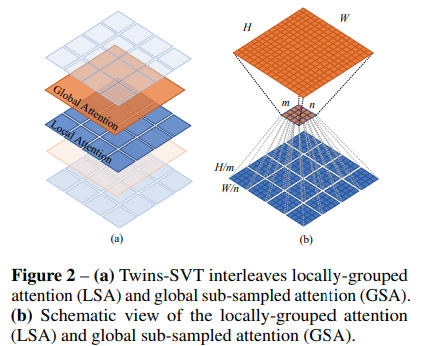This paper proposes mixing local and global attention, along with position encoding generator (proposed in CPVT) and global average pooling, to achieve the same results as Swin, without the extra complexity of shifted windows, CLS tokens, nor positional embeddings.

```import torch
from vit_pytorch.twins_svt import TwinsSVT

model = TwinsSVT(
num_classes = 1000,       # number of output classes
s1_emb_dim = 64,          # stage 1 - patch embedding projected dimension
s1_patch_size = 4,        # stage 1 - patch size for patch embedding
s1_local_patch_size = 7,  # stage 1 - patch size for local attention
s1_global_k = 7,          # stage 1 - global attention key / value reduction factor, defaults to 7 as specified in paper
s1_depth = 1,             # stage 1 - number of transformer blocks (local attn -> ff -> global attn -> ff)
s2_emb_dim = 128,         # stage 2 (same as above)
s2_patch_size = 2,
s2_local_patch_size = 7,
s2_global_k = 7,
s2_depth = 1,
s3_emb_dim = 256,         # stage 3 (same as above)
s3_patch_size = 2,
s3_local_patch_size = 7,
s3_global_k = 7,
s3_depth = 5,
s4_emb_dim = 512,         # stage 4 (same as above)
s4_patch_size = 2,
s4_local_patch_size = 7,
s4_global_k = 7,
s4_depth = 4,
peg_kernel_size = 3,      # positional encoding generator kernel size
dropout = 0.              # dropout
)

img = torch.randn(1, 3, 224, 224)

pred = model(img) # (1, 1000)```

## RegionViT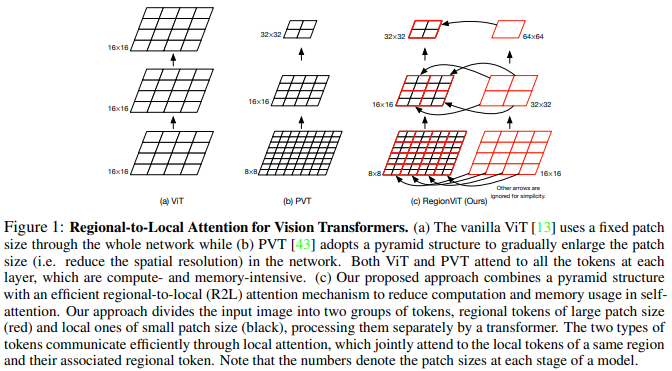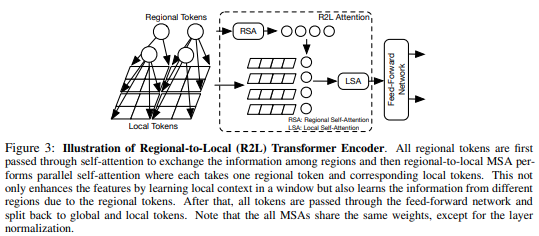This paper proposes to divide up the feature map into local regions, whereby the local tokens attend to each other. Each local region has its own regional token which then attends to all its local tokens, as well as other regional tokens.

You can use it as follows

```import torch
from vit_pytorch.regionvit import RegionViT

model = RegionViT(
dim = (64, 128, 256, 512),      # tuple of size 4, indicating dimension at each stage
depth = (2, 2, 8, 2),           # depth of the region to local transformer at each stage
window_size = 7,                # window size, which should be either 7 or 14
num_classes = 1000,             # number of output classes
tokenize_local_3_conv = False,  # whether to use a 3 layer convolution to encode the local tokens from the image. the paper uses this for the smaller models, but uses only 1 conv (set to False) for the larger models
use_peg = False,                # whether to use positional generating module. they used this for object detection for a boost in performance
)

img = torch.randn(1, 3, 224, 224)

pred = model(img) # (1, 1000)```

## CrossFormer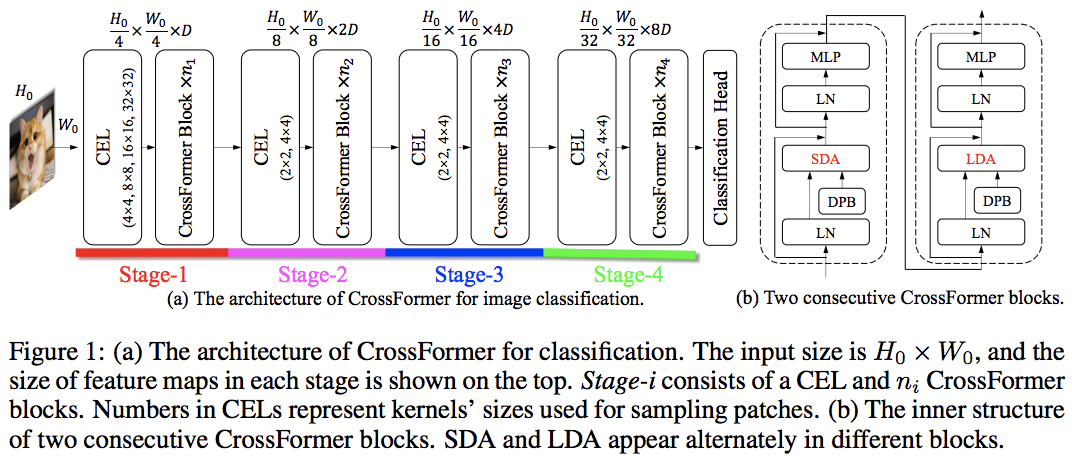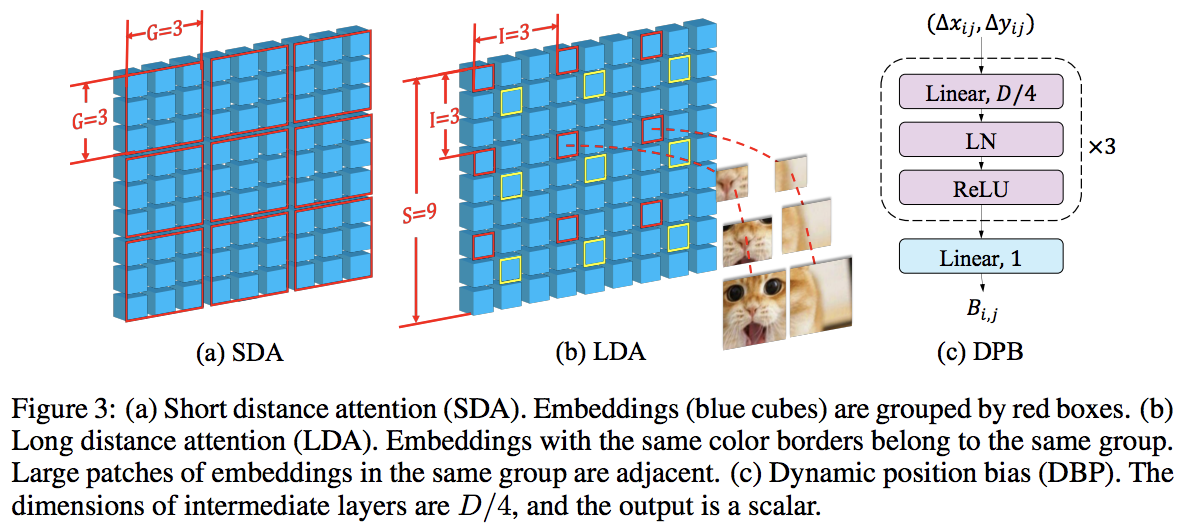This paper beats PVT and Swin using alternating local and global attention. The global attention is done across the windowing dimension for reduced complexity, much like the scheme used for axial attention.

They also have cross-scale embedding layer, which they shown to be a generic layer that can improve all vision transformers. Dynamic relative positional bias was also formulated to allow the net to generalize to images of greater resolution.

```import torch
from vit_pytorch.crossformer import CrossFormer

model = CrossFormer(
num_classes = 1000,                # number of output classes
dim = (64, 128, 256, 512),         # dimension at each stage
depth = (2, 2, 8, 2),              # depth of transformer at each stage
global_window_size = (8, 4, 2, 1), # global window sizes at each stage
local_window_size = 7,             # local window size (can be customized for each stage, but in paper, held constant at 7 for all stages)
)

img = torch.randn(1, 3, 224, 224)

pred = model(img) # (1, 1000)```

## ScalableViT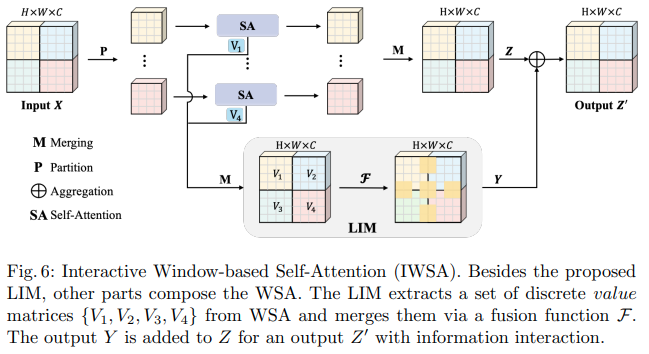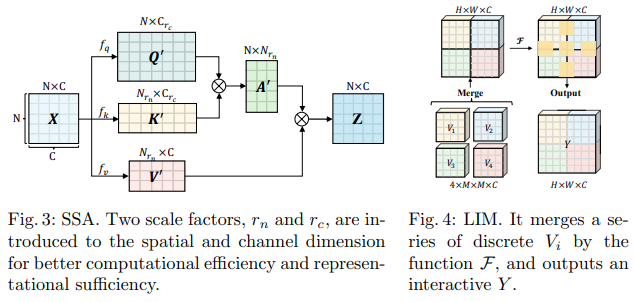This Bytedance AI paper proposes the Scalable Self Attention (SSA) and the Interactive Windowed Self Attention (IWSA) modules. The SSA alleviates the computation needed at earlier stages by reducing the key / value feature map by some factor (`reduction_factor`), while modulating the dimension of the queries and keys (`ssa_dim_key`). The IWSA performs self attention within local windows, similar to other vision transformer papers. However, they add a residual of the values, passed through a convolution of kernel size 3, which they named Local Interactive Module (LIM).

They make the claim in this paper that this scheme outperforms Swin Transformer, and also demonstrate competitive performance against Crossformer.

You can use it as follows (ex. ScalableViT-S)

```import torch
from vit_pytorch.scalable_vit import ScalableViT

model = ScalableViT(
num_classes = 1000,
dim = 64,                               # starting model dimension. at every stage, dimension is doubled
heads = (2, 4, 8, 16),                  # number of attention heads at each stage
depth = (2, 2, 20, 2),                  # number of transformer blocks at each stage
ssa_dim_key = (40, 40, 40, 32),         # the dimension of the attention keys (and queries) for SSA. in the paper, they represented this as a scale factor on the base dimension per key (ssa_dim_key / dim_key)
reduction_factor = (8, 4, 2, 1),        # downsampling of the key / values in SSA. in the paper, this was represented as (reduction_factor ** -2)
window_size = (64, 32, None, None),     # window size of the IWSA at each stage. None means no windowing needed
dropout = 0.1,                          # attention and feedforward dropout
)

img = torch.randn(1, 3, 256, 256)

preds = model(img) # (1, 1000)```

## SepViTAnother Bytedance AI paper, it proposes a depthwise-pointwise self-attention layer that seems largely inspired by mobilenet's depthwise-separable convolution. The most interesting aspect is the reuse of the feature map from the depthwise self-attention stage as the values for the pointwise self-attention, as shown in the diagram above.

I have decided to include only the version of `SepViT` with this specific self-attention layer, as the grouped attention layers are not remarkable nor novel, and the authors were not clear on how they treated the window tokens for the group self-attention layer. Besides, it seems like with `DSSA` layer alone, they were able to beat Swin.

ex. SepViT-Lite

```import torch
from vit_pytorch.sep_vit import SepViT

v = SepViT(
num_classes = 1000,
dim = 32,               # dimensions of first stage, which doubles every stage (32, 64, 128, 256) for SepViT-Lite
heads = (1, 2, 4, 8),   # number of heads per stage
depth = (1, 2, 6, 2),   # number of transformer blocks per stage
window_size = 7,        # window size of DSS Attention block
dropout = 0.1           # dropout
)

img = torch.randn(1, 3, 224, 224)

preds = v(img) # (1, 1000)```

## MaxViT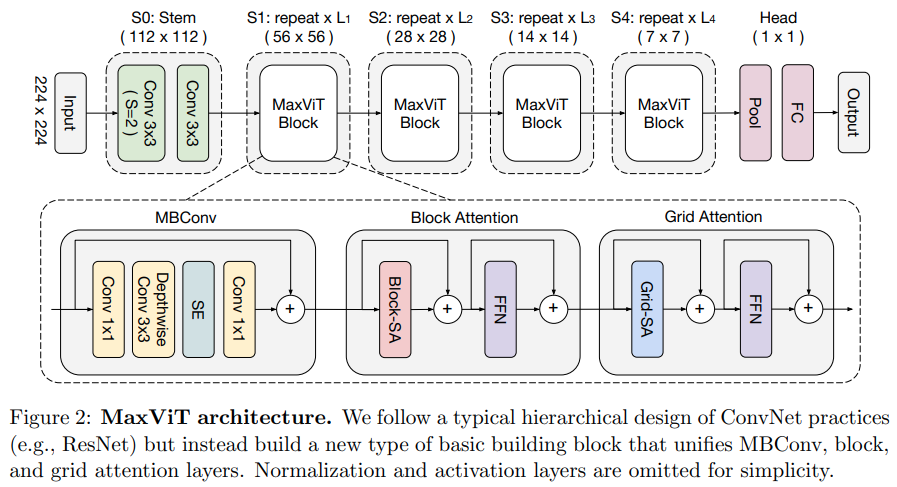This paper proposes a hybrid convolutional / attention network, using MBConv from the convolution side, and then block / grid axial sparse attention.

They also claim this specific vision transformer is good for generative models (GANs).

ex. MaxViT-S

```import torch
from vit_pytorch.max_vit import MaxViT

v = MaxViT(
num_classes = 1000,
dim_conv_stem = 64,               # dimension of the convolutional stem, would default to dimension of first layer if not specified
dim = 96,                         # dimension of first layer, doubles every layer
dim_head = 32,                    # dimension of attention heads, kept at 32 in paper
depth = (2, 2, 5, 2),             # number of MaxViT blocks per stage, which consists of MBConv, block-like attention, grid-like attention
window_size = 7,                  # window size for block and grids
mbconv_expansion_rate = 4,        # expansion rate of MBConv
mbconv_shrinkage_rate = 0.25,     # shrinkage rate of squeeze-excitation in MBConv
dropout = 0.1                     # dropout
)

img = torch.randn(2, 3, 224, 224)

preds = v(img) # (2, 1000)```

## NesT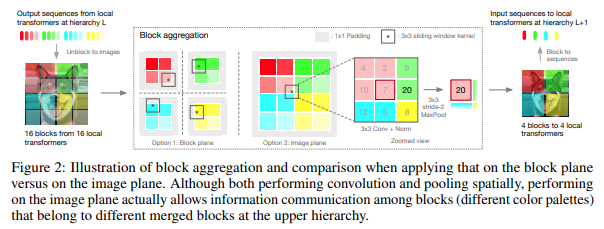This paper decided to process the image in hierarchical stages, with attention only within tokens of local blocks, which aggregate as it moves up the heirarchy. The aggregation is done in the image plane, and contains a convolution and subsequent maxpool to allow it to pass information across the boundary.

You can use it with the following code (ex. NesT-T)

```import torch
from vit_pytorch.nest import NesT

nest = NesT(
image_size = 224,
patch_size = 4,
dim = 96,
num_hierarchies = 3,        # number of hierarchies
block_repeats = (2, 2, 8),  # the number of transformer blocks at each heirarchy, starting from the bottom
num_classes = 1000
)

img = torch.randn(1, 3, 224, 224)

pred = nest(img) # (1, 1000)```

## MobileViT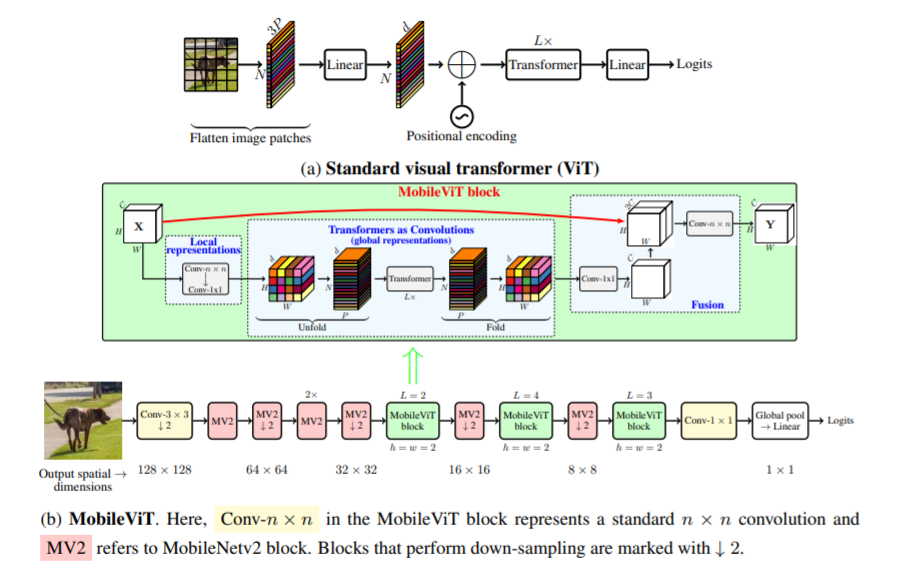This paper introduce MobileViT, a light-weight and general purpose vision transformer for mobile devices. MobileViT presents a different perspective for the global processing of information with transformers.

You can use it with the following code (ex. mobilevit_xs)

```import torch
from vit_pytorch.mobile_vit import MobileViT

mbvit_xs = MobileViT(
image_size = (256, 256),
dims = [96, 120, 144],
channels = [16, 32, 48, 48, 64, 64, 80, 80, 96, 96, 384],
num_classes = 1000
)

img = torch.randn(1, 3, 256, 256)

pred = mbvit_xs(img) # (1, 1000)```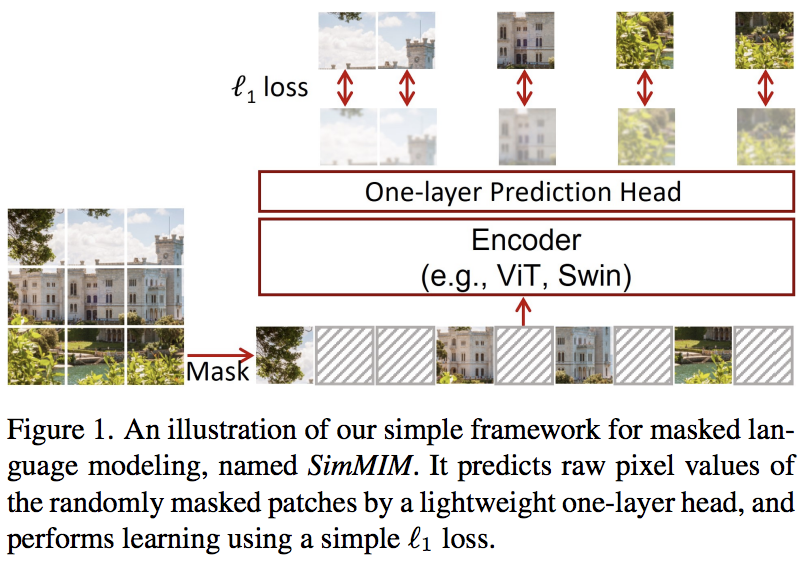This paper proposes a simple masked image modeling (SimMIM) scheme, using only a linear projection off the masked tokens into pixel space followed by an L1 loss with the pixel values of the masked patches. Results are competitive with other more complicated approaches.

You can use this as follows

```import torch
from vit_pytorch import ViT
from vit_pytorch.simmim import SimMIM

v = ViT(
image_size = 256,
patch_size = 32,
num_classes = 1000,
dim = 1024,
depth = 6,
mlp_dim = 2048
)

mim = SimMIM(
encoder = v,
masking_ratio = 0.5  # they found 50% to yield the best results
)

images = torch.randn(8, 3, 256, 256)

loss = mim(images)
loss.backward()

# that's all!
# do the above in a for loop many times with a lot of images and your vision transformer will learn

torch.save(v.state_dict(), './trained-vit.pt')```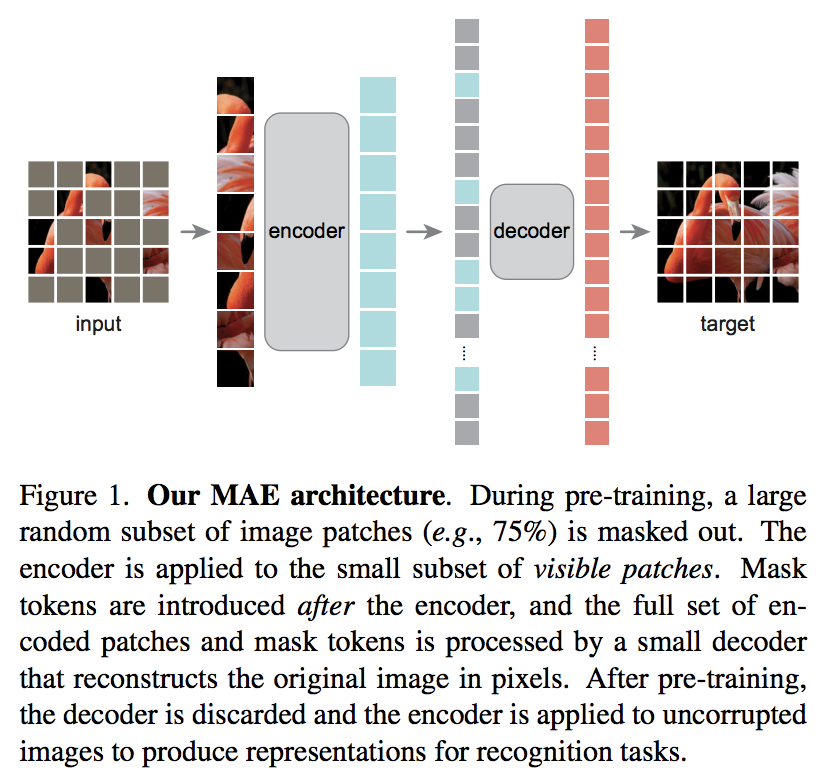A new Kaiming He paper proposes a simple autoencoder scheme where the vision transformer attends to a set of unmasked patches, and a smaller decoder tries to reconstruct the masked pixel values.

AI Coffeebreak with Letitia

You can use it with the following code

```import torch
from vit_pytorch import ViT, MAE

v = ViT(
image_size = 256,
patch_size = 32,
num_classes = 1000,
dim = 1024,
depth = 6,
mlp_dim = 2048
)

mae = MAE(
encoder = v,
decoder_dim = 512,      # paper showed good results with just 512
decoder_depth = 6       # anywhere from 1 to 8
)

images = torch.randn(8, 3, 256, 256)

loss = mae(images)
loss.backward()

# that's all!
# do the above in a for loop many times with a lot of images and your vision transformer will learn

# save your improved vision transformer
torch.save(v.state_dict(), './trained-vit.pt')```

Thanks to Zach, you can train using the original masked patch prediction task presented in the paper, with the following code.

```import torch
from vit_pytorch import ViT
from vit_pytorch.mpp import MPP

model = ViT(
image_size=256,
patch_size=32,
num_classes=1000,
dim=1024,
depth=6,
mlp_dim=2048,
dropout=0.1,
emb_dropout=0.1
)

mpp_trainer = MPP(
transformer=model,
patch_size=32,
dim=1024,
random_patch_prob=0.30,  # probability of randomly replacing a token being used for mpp
replace_prob=0.50,       # probability of replacing a token being used for mpp with the mask token
)

def sample_unlabelled_images():

for _ in range(100):
images = sample_unlabelled_images()
loss = mpp_trainer(images)
loss.backward()
opt.step()

torch.save(model.state_dict(), './pretrained-net.pt')```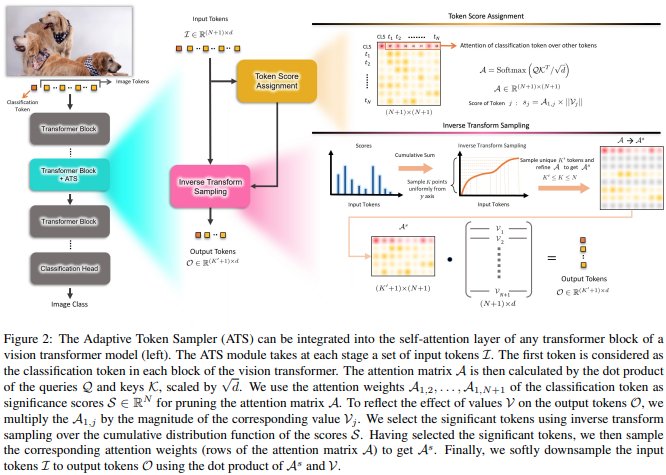This paper proposes to use the CLS attention scores, re-weighed by the norms of the value heads, as means to discard unimportant tokens at different layers.

```import torch
from vit_pytorch.ats_vit import ViT

v = ViT(
image_size = 256,
patch_size = 16,
num_classes = 1000,
dim = 1024,
depth = 6,
max_tokens_per_depth = (256, 128, 64, 32, 16, 8), # a tuple that denotes the maximum number of tokens that any given layer should have. if the layer has greater than this amount, it will undergo adaptive token sampling
mlp_dim = 2048,
dropout = 0.1,
emb_dropout = 0.1
)

img = torch.randn(4, 3, 256, 256)

preds = v(img) # (4, 1000)

# you can also get a list of the final sampled patch ids
# a value of -1 denotes padding

preds, token_ids = v(img, return_sampled_token_ids = True) # (4, 1000), (4, <=8)```

## Patch Merger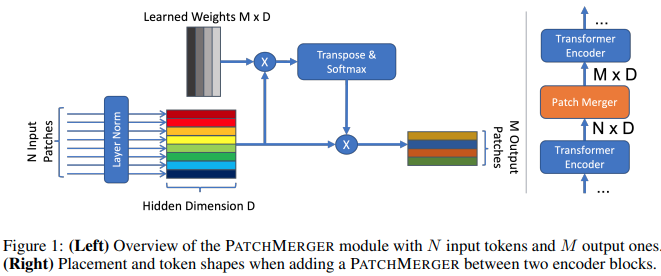This paper proposes a simple module (Patch Merger) for reducing the number of tokens at any layer of a vision transformer without sacrificing performance.

```import torch
from vit_pytorch.vit_with_patch_merger import ViT

v = ViT(
image_size = 256,
patch_size = 16,
num_classes = 1000,
dim = 1024,
depth = 12,
patch_merge_layer = 6,        # at which transformer layer to do patch merging
patch_merge_num_tokens = 8,   # the output number of tokens from the patch merge
mlp_dim = 2048,
dropout = 0.1,
emb_dropout = 0.1
)

img = torch.randn(4, 3, 256, 256)

preds = v(img) # (4, 1000)```

One can also use the `PatchMerger` module by itself

```import torch
from vit_pytorch.vit_with_patch_merger import PatchMerger

merger = PatchMerger(
dim = 1024,
num_tokens_out = 8   # output number of tokens
)

features = torch.randn(4, 256, 1024) # (batch, num tokens, dimension)

out = merger(features) # (4, 8, 1024)```

## Vision Transformer for Small Datasets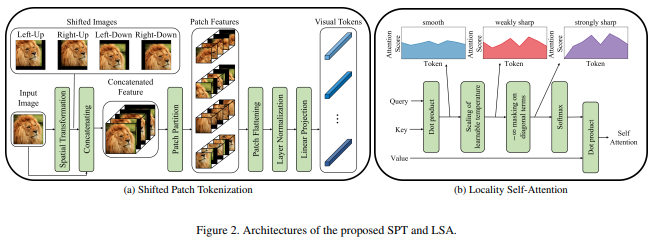This paper proposes a new image to patch function that incorporates shifts of the image, before normalizing and dividing the image into patches. I have found shifting to be extremely helpful in some other transformers work, so decided to include this for further explorations. It also includes the `LSA` with the learned temperature and masking out of a token's attention to itself.

You can use as follows:

```import torch
from vit_pytorch.vit_for_small_dataset import ViT

v = ViT(
image_size = 256,
patch_size = 16,
num_classes = 1000,
dim = 1024,
depth = 6,
mlp_dim = 2048,
dropout = 0.1,
emb_dropout = 0.1
)

img = torch.randn(4, 3, 256, 256)

preds = v(img) # (1, 1000)```

You can also use the `SPT` from this paper as a standalone module

```import torch
from vit_pytorch.vit_for_small_dataset import SPT

spt = SPT(
dim = 1024,
patch_size = 16,
channels = 3
)

img = torch.randn(4, 3, 256, 256)

tokens = spt(img) # (4, 256, 1024)```

## Parallel ViT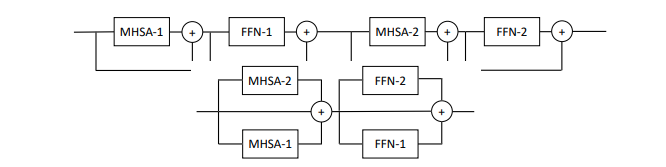This paper propose parallelizing multiple attention and feedforward blocks per layer (2 blocks), claiming that it is easier to train without loss of performance.

You can try this variant as follows

```import torch
from vit_pytorch.parallel_vit import ViT

v = ViT(
image_size = 256,
patch_size = 16,
num_classes = 1000,
dim = 1024,
depth = 6,
mlp_dim = 2048,
num_parallel_branches = 2,  # in paper, they claimed 2 was optimal
dropout = 0.1,
emb_dropout = 0.1
)

img = torch.randn(4, 3, 256, 256)

preds = v(img) # (4, 1000)```

## Learnable Memory ViT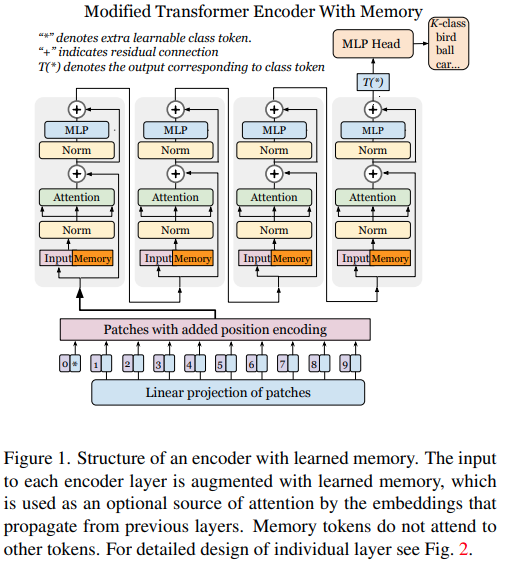This paper shows that adding learnable memory tokens at each layer of a vision transformer can greatly enhance fine-tuning results (in addition to learnable task specific CLS token and adapter head).

You can use this with a specially modified `ViT` as follows

```import torch

# normal base ViT

v = ViT(
image_size = 256,
patch_size = 16,
num_classes = 1000,
dim = 1024,
depth = 6,
mlp_dim = 2048,
dropout = 0.1,
emb_dropout = 0.1
)

img = torch.randn(4, 3, 256, 256)
logits = v(img) # (4, 1000)

# do your usual training with ViT
# ...

# then, to finetune, just pass the ViT into the Adapter class
# you can do this for multiple Adapters, as shown below

vit = v,
num_classes = 2,               # number of output classes for this specific task
num_memories_per_layer = 5     # number of learnable memories per layer, 10 was sufficient in paper
)

logits1 = adapter1(img) # (4, 2) - predict 2 classes off frozen ViT backbone with learnable memories and task specific head

# yet another task to finetune on, this time with 4 classes

vit = v,
num_classes = 4,
num_memories_per_layer = 10
)

logits2 = adapter2(img) # (4, 4) - predict 4 classes off frozen ViT backbone with learnable memories and task specific head```

## Dino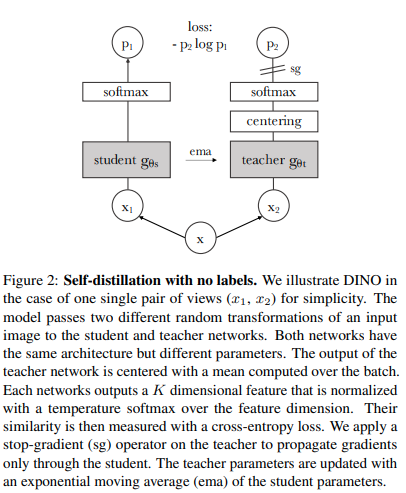You can train `ViT` with the recent SOTA self-supervised learning technique, Dino, with the following code.

Yannic Kilcher video

```import torch
from vit_pytorch import ViT, Dino

model = ViT(
image_size = 256,
patch_size = 32,
num_classes = 1000,
dim = 1024,
depth = 6,
mlp_dim = 2048
)

learner = Dino(
model,
image_size = 256,
hidden_layer = 'to_latent',        # hidden layer name or index, from which to extract the embedding
projection_hidden_size = 256,      # projector network hidden dimension
projection_layers = 4,             # number of layers in projection network
num_classes_K = 65336,             # output logits dimensions (referenced as K in paper)
student_temp = 0.9,                # student temperature
teacher_temp = 0.04,               # teacher temperature, needs to be annealed from 0.04 to 0.07 over 30 epochs
local_upper_crop_scale = 0.4,      # upper bound for local crop - 0.4 was recommended in the paper
global_lower_crop_scale = 0.5,     # lower bound for global crop - 0.5 was recommended in the paper
moving_average_decay = 0.9,        # moving average of encoder - paper showed anywhere from 0.9 to 0.999 was ok
center_moving_average_decay = 0.9, # moving average of teacher centers - paper showed anywhere from 0.9 to 0.999 was ok
)

opt = torch.optim.Adam(learner.parameters(), lr = 3e-4)

def sample_unlabelled_images():

for _ in range(100):
images = sample_unlabelled_images()
loss = learner(images)
loss.backward()
opt.step()
learner.update_moving_average() # update moving average of teacher encoder and teacher centers

torch.save(model.state_dict(), './pretrained-net.pt')```

## EsViT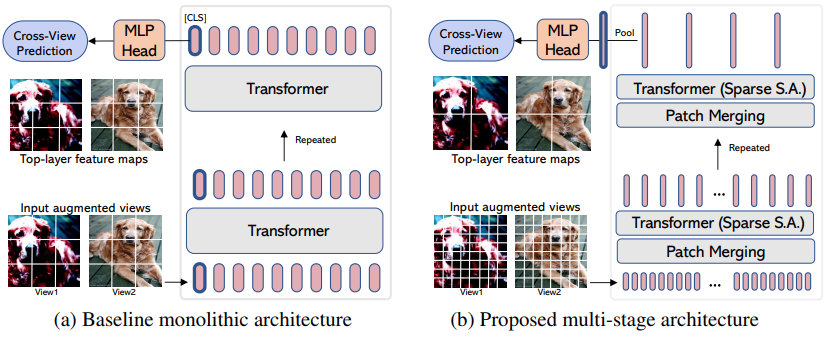`EsViT` is a variant of Dino (from above) re-engineered to support efficient `ViT`s with patch merging / downsampling by taking into an account an extra regional loss between the augmented views. To quote the abstract, it `outperforms its supervised counterpart on 17 out of 18 datasets` at 3 times higher throughput.

Even though it is named as though it were a new `ViT` variant, it actually is just a strategy for training any multistage `ViT` (in the paper, they focused on Swin). The example below will show how to use it with `CvT`. You'll need to set the `hidden_layer` to the name of the layer within your efficient ViT that outputs the non-average pooled visual representations, just before the global pooling and projection to logits.

```import torch
from vit_pytorch.cvt import CvT
from vit_pytorch.es_vit import EsViTTrainer

cvt = CvT(
num_classes = 1000,
s1_emb_dim = 64,
s1_emb_kernel = 7,
s1_emb_stride = 4,
s1_proj_kernel = 3,
s1_kv_proj_stride = 2,
s1_depth = 1,
s1_mlp_mult = 4,
s2_emb_dim = 192,
s2_emb_kernel = 3,
s2_emb_stride = 2,
s2_proj_kernel = 3,
s2_kv_proj_stride = 2,
s2_depth = 2,
s2_mlp_mult = 4,
s3_emb_dim = 384,
s3_emb_kernel = 3,
s3_emb_stride = 2,
s3_proj_kernel = 3,
s3_kv_proj_stride = 2,
s3_depth = 10,
s3_mlp_mult = 4,
dropout = 0.
)

learner = EsViTTrainer(
cvt,
image_size = 256,
hidden_layer = 'layers',           # hidden layer name or index, from which to extract the embedding
projection_hidden_size = 256,      # projector network hidden dimension
projection_layers = 4,             # number of layers in projection network
num_classes_K = 65336,             # output logits dimensions (referenced as K in paper)
student_temp = 0.9,                # student temperature
teacher_temp = 0.04,               # teacher temperature, needs to be annealed from 0.04 to 0.07 over 30 epochs
local_upper_crop_scale = 0.4,      # upper bound for local crop - 0.4 was recommended in the paper
global_lower_crop_scale = 0.5,     # lower bound for global crop - 0.5 was recommended in the paper
moving_average_decay = 0.9,        # moving average of encoder - paper showed anywhere from 0.9 to 0.999 was ok
center_moving_average_decay = 0.9, # moving average of teacher centers - paper showed anywhere from 0.9 to 0.999 was ok
)

opt = torch.optim.AdamW(learner.parameters(), lr = 3e-4)

def sample_unlabelled_images():

for _ in range(1000):
images = sample_unlabelled_images()
loss = learner(images)
loss.backward()
opt.step()
learner.update_moving_average() # update moving average of teacher encoder and teacher centers

torch.save(cvt.state_dict(), './pretrained-net.pt')```

## Accessing Attention

If you would like to visualize the attention weights (post-softmax) for your research, just follow the procedure below

```import torch
from vit_pytorch.vit import ViT

v = ViT(
image_size = 256,
patch_size = 32,
num_classes = 1000,
dim = 1024,
depth = 6,
mlp_dim = 2048,
dropout = 0.1,
emb_dropout = 0.1
)

# import Recorder and wrap the ViT

from vit_pytorch.recorder import Recorder
v = Recorder(v)

# forward pass now returns predictions and the attention maps

img = torch.randn(1, 3, 256, 256)
preds, attns = v(img)

# there is one extra patch due to the CLS token

attns # (1, 6, 16, 65, 65) - (batch x layers x heads x patch x patch)```

to cleanup the class and the hooks once you have collected enough data

`v = v.eject()  # wrapper is discarded and original ViT instance is returned`

## Accessing Embeddings

You can similarly access the embeddings with the `Extractor` wrapper

```import torch
from vit_pytorch.vit import ViT

v = ViT(
image_size = 256,
patch_size = 32,
num_classes = 1000,
dim = 1024,
depth = 6,
mlp_dim = 2048,
dropout = 0.1,
emb_dropout = 0.1
)

# import Recorder and wrap the ViT

from vit_pytorch.extractor import Extractor
v = Extractor(v)

# forward pass now returns predictions and the attention maps

img = torch.randn(1, 3, 256, 256)
logits, embeddings = v(img)

# there is one extra token due to the CLS token

embeddings # (1, 65, 1024) - (batch x patches x model dim)```

## Research Ideas

### Efficient Attention

There may be some coming from computer vision who think attention still suffers from quadratic costs. Fortunately, we have a lot of new techniques that may help. This repository offers a way for you to plugin your own sparse attention transformer.

An example with Nystromformer

`\$ pip install nystrom-attention`
```import torch
from vit_pytorch.efficient import ViT
from nystrom_attention import Nystromformer

efficient_transformer = Nystromformer(
dim = 512,
depth = 12,
num_landmarks = 256
)

v = ViT(
dim = 512,
image_size = 2048,
patch_size = 32,
num_classes = 1000,
transformer = efficient_transformer
)

img = torch.randn(1, 3, 2048, 2048) # your high resolution picture
v(img) # (1, 1000)```

Other sparse attention frameworks I would highly recommend is Routing Transformer or Sinkhorn Transformer

### Combining with other Transformer improvements

This paper purposely used the most vanilla of attention networks to make a statement. If you would like to use some of the latest improvements for attention nets, please use the `Encoder` from this repository.

ex.

`\$ pip install x-transformers`
```import torch
from vit_pytorch.efficient import ViT
from x_transformers import Encoder

v = ViT(
dim = 512,
image_size = 224,
patch_size = 16,
num_classes = 1000,
transformer = Encoder(
dim = 512,                  # set to be the same as the wrapper
depth = 12,
ff_glu = True,              # ex. feed forward GLU variant https://arxiv.org/abs/2002.05202
residual_attn = True        # ex. residual attention https://arxiv.org/abs/2012.11747
)
)

img = torch.randn(1, 3, 224, 224)
v(img) # (1, 1000)```

## FAQ

• How do I pass in non-square images?

You can already pass in non-square images - you just have to make sure your height and width is less than or equal to the `image_size`, and both divisible by the `patch_size`

ex.

```import torch
from vit_pytorch import ViT

v = ViT(
image_size = 256,
patch_size = 32,
num_classes = 1000,
dim = 1024,
depth = 6,
mlp_dim = 2048,
dropout = 0.1,
emb_dropout = 0.1
)

img = torch.randn(1, 3, 256, 128) # <-- not a square

preds = v(img) # (1, 1000)```
• How do I pass in non-square patches?
```import torch
from vit_pytorch import ViT

v = ViT(
num_classes = 1000,
image_size = (256, 128),  # image size is a tuple of (height, width)
patch_size = (32, 16),    # patch size is a tuple of (height, width)
dim = 1024,
depth = 6,
mlp_dim = 2048,
dropout = 0.1,
emb_dropout = 0.1
)

img = torch.randn(1, 3, 256, 128)

preds = v(img)```

## Resources

Coming from computer vision and new to transformers? Here are some resources that greatly accelerated my learning.

1. Illustrated Transformer - Jay Alammar

2. Transformers from Scratch - Peter Bloem

3. The Annotated Transformer - Harvard NLP

## Citations